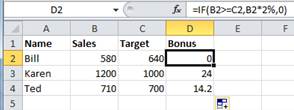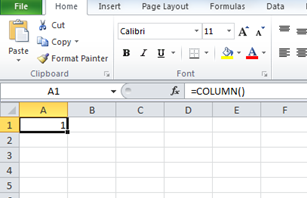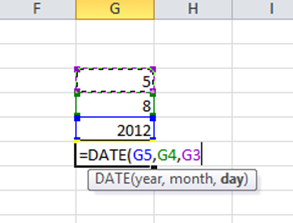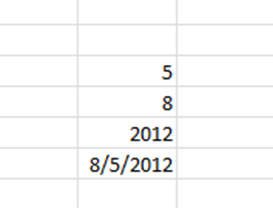## Tuesday, November 13, 2012

### How to add Chitika on the bloger

Over time, I’ve noticed that this question pops up quite a bit:
If my domain is not listed on the “Approved Domains” page, does that mean my ads aren’t working on that site?
To add sites to your approved domains list, just go to the “Domains” tab after logging into your account, and submit the sites in the white box at the top of the page:

## Friday, August 24, 2012

### Using IF True/False in excel

Provided that you remember that True value always precedes False value, these two values can be almost anything. If desired, a simple number could be returned, a calculation performed, or even a piece of text entered. Also, the type of data entered can vary depending on whether it is a true or false result. You may want a calculation if the logical test is true, but a message displayed if false. (Remember that test to be included in functions should be enclosed in quotes). Taking the same logical test mentioned above, if the sales figure meets or exceeds the target, a Bonus is calculated (e.g 2% of sales). If not, no bonus is calculated so a value of zero is returned. The IF statement in column D of the Example reads as follows;

=IF(B2>=C2,B2*2%,0)You may, alternatively, want to see a message saying “ No Bonus”. In this case, the true value will remain the same and the false value will be the text string “No Bonus” ;

=IF(B2>=C2,B2*2%,"No Bonus")

## Sunday, August 5, 2012

### Column Function

In Excel, the Column function returns the column number of a cell reference.
The syntax for the Column function is:
Column( reference )
reference is optional. It is a reference to a cell or range of cells.
Note:
If the reference parameter is omitted, then the Column function assumes that the reference is the cell address in which the Column function has been entered in.For example, the Column function used in the picture above returns 1 because the Column function has been entered in cell A1. Therefore, it assumes the following formula:
=Column(A1)
Applies To:
• Excel 2010
For Example:
Let's take a look at an example:
 =Column(B4) would return 2 =Column(B4:B6) would return 2

### Date & Time Functions in Excel

In Excel, the Date function returns the serial number of a date.
The syntax for the Date function is:
Date( year, month, day )
year is a number that is between one and four digits.
• If the year is between 0 and 1899, the year value is added to 1900 to determine the year.
• If the year is between 1900 and 9999, the Date function uses the year value as the year.
• If the year is greater than 9999, the Date function will return the #NUM! error.
month is a number representing the month value. If the month value is greater than 12, the Date function adds that number of months to the first month of the year specified in the year parameter.
day is a number representing the day value. If the day value is greater than the number of days in the month specified, the Date function add that number of days to the first day in the month specified in the month parameter.
Applies To:
• Excel 2010
For Example:
Let's take a look at an exampleThis result below